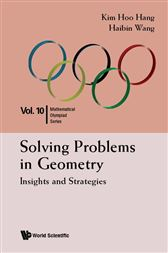# Solving Problems in Geometry

## Series: Mathematical Olympiad Series (No. 10)US\$27.00
US\$24.57
###### ISBNs
• 9789814590723
• 9789814583763
-->

This new volume of the Mathematical Olympiad Series focuses on the topic of geometry. Basic and advanced theorems commonly seen in Mathematical Olympiad are introduced and illustrated with plenty of examples. Special techniques in solving various types of geometrical problems are also introduced, while the authors elaborate extensively on how to acquire an insight and develop strategies in tackling difficult geometrical problems.

This book is suitable for any reader with elementary geometrical knowledge at the lower secondary level. Each chapter includes sufficient scaffolding and is comprehensive enough for the purpose of self-study. Readers who complete the chapters on the basic theorems and techniques would acquire a good foundation in geometry and may attempt to solve many geometrical problems in various mathematical competitions. Meanwhile, experienced contestants in Mathematical Olympiad competitions will find a large collection of problems pitched at competitions at the international level, with opportunities to practise and sharpen their problem-solving skills in geometry.

-->
###### ISBNs
• 9789814590723
• 9789814583763
###### ISBNs
• 9789814590723
• 9789814583763